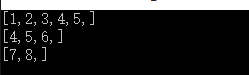# 【BAT经典算法面试题系列】求和为n的连续正整数

#include <iostream>
using namespace std;

void printResult(int start,int end)
{
cout<<"[";
for(int i=start;i<=end;i++)
cout<<i<<",";
cout<<"]"<<endl;
}

void findSumIsN(int n)
{
int start=1,end=2;
int sum=start+end;;
while(start<(n+1)/2)
{

if(sum==n)
{
printResult(start,end);
}
while(sum<n)
{
end++;
sum+=end;
if(sum==n)
{
printResult(start,end);

}
}
sum-=start;
start++;

}
}

int main(int argc, char** argv) {

findSumIsN(15);
return 0;
}